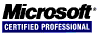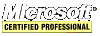## Data Compression Algorithm Study in C/C++

This code is a study of data compression algorithms. LZW 15 Bit Variable Rate Encoder, LZW 12 Bit Encoder, LZSS Encoder, static order 0 model with Huffman coding, adaptive order 1 model with arithmetic coding, fixed order 0 model with arithmetic coding, and adaptive Huffman coding compression algorithms are covered. Download it from here: DATACOMPRESSION.ZIP

``````
/************************** Start of LZW15V.C *************************
*
* This is the LZW module which implements a more powerful version
* of the algorithm.	This version of the program has three major
* improvements over LZW12.C.	First, it expands the maximum code size
* to 15 bits.	Second, it starts encoding with 9 bit codes, working
* its way up in bit size only as necessary.	Finally, it flushes the
* dictionary when done.
*
*
*/
#include <stdio.h>
#include <stdlib.h>
#include <string.h>
#include <windows.h>
#include "errhand.h"
#include "bitio.h"

/*
* Constants used throughout the program.	BITS defines the maximum
* number of bits that can be used in the output code.	TABLE_SIZE defines
* the size of the dictionary table.	TABLE_BANKS are the number of
* 256 element dictionary pages needed.	The code defines should be
* self-explanatory.
*/

#define BITS						 15
#define MAX_CODE					 ( ( 1 << BITS ) - 1 )
#define TABLE_SIZE				 35023L
#define TABLE_BANKS				( ( TABLE_SIZE >> 8 ) + 1 )
#define END_OF_STREAM				256
#define BUMP_CODE					257
#define FLUSH_CODE				 258
#define FIRST_CODE				 259
#define UNUSED					 -1

/*
* Local prototypes.
*/

unsigned int find_child_node( int parent_code, int child_character );
unsigned int decode_string( unsigned int offset, unsigned int code );

//char *CompressionName = "LZW 15 Bit Variable Rate Encoder";
//char *Usage			 = "in-file out-file\n\n";

/*
* This data structure defines the dictionary.	Each entry in the dictionary
* has a code value.	This is the code emitted by the compressor.	Each
* code is actually made up of two pieces:	a parent_code, and a
* character.	Code values of less than 256 are actually plain
* text codes.
*
* Note that in order to handle 16 bit segmented compilers, such as most
* of the MS-DOS compilers, it was necessary to break up the dictionary
* into a table of smaller dictionary pointers.	Every reference to the
* dictionary was replaced by a macro that did a pointer dereference first.
* By breaking up the index along byte boundaries we should be as efficient
* as possible.
*/

struct dictionary {
int code_value;
int parent_code;
char character;
} *dict[ TABLE_BANKS ];

#define DICT( i ) dict[ i >> 8 ][ i & 0xff ]

/*
* Other global data structures.	The decode_stack is used to reverse
* strings that come out of the tree during decoding.	next_code is the
* next code to be added to the dictionary, both during compression and
* decompression.	current_code_bits defines how many bits are currently
* being used for output, and next_bump_code defines the code that will
* trigger the next jump in word size.
*/

static char decode_stack[ TABLE_SIZE ];
unsigned int next_code;
int current_code_bits;
unsigned int next_bump_code;

/*
* This routine is used to initialize the dictionary, both when the
* compressor or decompressor first starts up, and also when a flush
* code comes in.	Note that even thought the decompressor sets all
* the code_value elements to UNUSED, it doesn't really need to.
*/

void InitializeDictionary()
{
unsigned int i;

for ( i = 0 ; i < TABLE_SIZE ; i++ )
DICT( i ).code_value = UNUSED;
next_code = FIRST_CODE;
current_code_bits = 9;
next_bump_code = 511;
}

/*
* This routine allocates the dictionary.	Since the total size of the
* dictionary is much larger than 64K, it can't be allocated as a single
* object.	Instead, it is allocated as a set of pointers to smaller
* dictionary objects.	The special DICT() macro is used to translate
* indices into pairs of references.
*/

void InitializeStorage()
{
int i;

for ( i = 0 ; i < TABLE_BANKS ; i++ ) {
dict[ i ] = (struct dictionary *) calloc( 256, sizeof ( struct dictionary ) );
if ( dict[ i ] == NULL )
fatal_error( "Error allocating dictionary space" );
}
}

/*
* The compressor is short and simple.	It reads in new symbols one
* at a time from the input file.	It then	checks to see if the
* combination of the current symbol and the current code are already
* defined in the dictionary.	If they are not, they are added to the
* dictionary, and we start over with a new one symbol code.	If they
* are, the code for the combination of the code and character becomes
* our new code.	Note that in this enhanced version of LZW, the
* encoder needs to check the codes for boundary conditions.
*/

int CompressFile_lzw_15_bit_variable_rate_encoder(FILE *input,BIT_FILE *output,int argc,char *argv[])
{
int character;
int string_code;
unsigned int index;

DWORD milliseconds = GetTickCount();
InitializeStorage();
InitializeDictionary();
if ( ( string_code = getc( input ) ) == EOF )
string_code = END_OF_STREAM;
while ( ( character = getc( input ) ) != EOF ) {
index = find_child_node( string_code, character );
if ( DICT( index ).code_value != - 1 )
string_code = DICT( index ).code_value;
else {
DICT( index ).code_value = next_code++;
DICT( index ).parent_code = string_code;
DICT( index ).character = (char) character;
OutputBits( output, (unsigned long) string_code, current_code_bits );
string_code = character;
if ( next_code > MAX_CODE ) {
OutputBits( output, (unsigned long) FLUSH_CODE, current_code_bits );
InitializeDictionary();
} else if ( next_code > next_bump_code ) {
OutputBits( output, (unsigned long) BUMP_CODE, current_code_bits );
current_code_bits++;
next_bump_code <<= 1;
next_bump_code |= 1;
//putc( 'B', stdout );
}
}
}
OutputBits( output, (unsigned long) string_code, current_code_bits );
OutputBits( output, (unsigned long) END_OF_STREAM, current_code_bits);
return (GetTickCount() - milliseconds);
}

/*
* The file expander operates much like the encoder.	It has to
* read in codes, the convert the codes to a string of characters.
* The only catch in the whole operation occurs when the encoder
* encounters a CHAR+STRING+CHAR+STRING+CHAR sequence.	When this
* occurs, the encoder outputs a code that is not presently defined
* in the table.	This is handled as an exception.	All of the special
* input codes are handled in various ways.
*/

int ExpandFile_lzw_15_bit_variable_rate_encoder(BIT_FILE *input, FILE *output, int argc, char *argv[])
{
unsigned int new_code;
unsigned int old_code;
int character;
unsigned int count;

DWORD milliseconds = GetTickCount();
InitializeStorage();
for ( ; ; ) {
InitializeDictionary();
old_code = (unsigned int) InputBits( input, current_code_bits );
if ( old_code == END_OF_STREAM )
return (GetTickCount() - milliseconds);
character = old_code;
putc( old_code, output );
for ( ; ; ) {
new_code = (unsigned int) InputBits( input, current_code_bits );
if ( new_code == END_OF_STREAM )
return (GetTickCount() - milliseconds);
if ( new_code == FLUSH_CODE )
break;
if ( new_code == BUMP_CODE ) {
current_code_bits++;
continue;
}
if ( new_code >= next_code ) {
decode_stack[ 0 ] = (char) character;
count = decode_string( 1, old_code );
}
else
count = decode_string( 0, new_code );
character = decode_stack[ count - 1 ];
while ( count > 0 )
putc( decode_stack[ --count ], output );
DICT( next_code ).parent_code = old_code;
DICT( next_code ).character = (char) character;
next_code++;
old_code = new_code;
}
}
return (GetTickCount() - milliseconds);
}

/*
* This hashing routine is responsible for finding the table location
* for a string/character combination.	The table index is created
* by using an exclusive OR combination of the prefix and character.
* This code also has to check for collisions, and handles them by
* jumping around in the table.
*/

static unsigned int find_child_node(int parent_code,int child_character)
{
unsigned int index;
unsigned int offset;

index = ( child_character << ( BITS - 8 ) ) ^ parent_code;
if ( index == 0 )
offset = 1;
else
offset = TABLE_SIZE - index;
for ( ; ; ) {
if ( DICT( index ).code_value == UNUSED )
return( (unsigned int) index );
if ( DICT( index ).parent_code == parent_code &&
DICT( index ).character == (char) child_character )
return( index );
if ( (int) index >= offset )
index -= offset;
else
index += TABLE_SIZE - offset;
}
}

/*
* This routine decodes a string from the dictionary, and stores it
* in the decode_stack data structure.	It returns a count to the
* calling program of how many characters were placed in the stack.
*/

static unsigned int decode_string(unsigned int count, unsigned int code)
{
while ( code > 255 ) {
decode_stack[ count++ ] = DICT( code ).character;
code = DICT( code ).parent_code;
}
decode_stack[ count++ ] = (char) code;
return( count );
}

/************************** End of LZW15V.C *************************/
``````